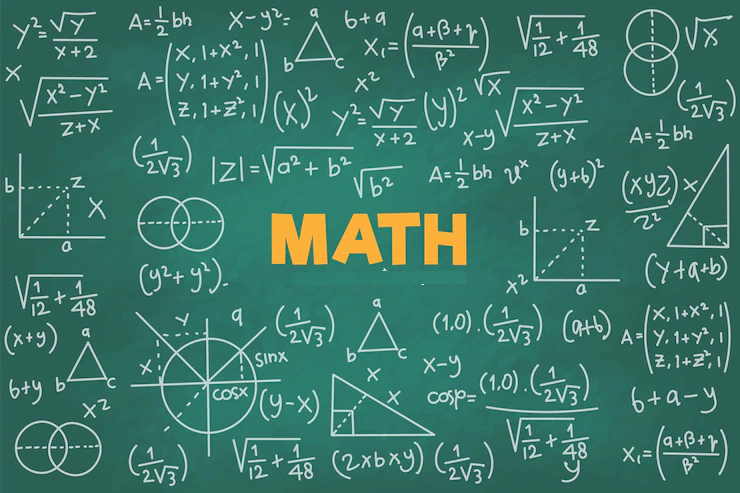# IB Math Applications and Interpretations

## Explore the Practical World of IB Math Applications and Interpretations

"Mathematics is not only a language of numbers but also a powerful tool for solving real-world problems and making sense of the world around us." - UnknownWelcome to the captivating realm of IB Math Applications and Interpretations, where you will embark on an exciting journey into the practical applications of mathematics in various contexts. This course offers a comprehensive exploration of the principles, techniques, and real-world applications of mathematics.

Course Overview:

1. Number and Algebra
2. Descriptive Statistics
3. Mathematical Models
4. Probability
5. Statistical Applications
6. Financial Mathematics
7. Networks and Decision Mathematics
8. Exam Preparation and Review

Whether you have a passion for analyzing data, making informed decisions, or understanding financial concepts, our IB Math Applications and Interpretations program offers a dynamic and rigorous study of the subject, enabling students to develop their mathematical skills, critical thinking, and problem-solving abilities.

Course Topics:

1. Number and Algebra: Explore number systems, algebraic techniques, and the application of algebra in solving equations and inequalities.

2. Descriptive Statistics: Analyze data sets, explore measures of central tendency and dispersion, and interpret statistical graphs and charts.

3. Mathematical Models: Investigate the use of mathematical models to represent real-world situations, making predictions and analyzing trends.

4. Probability: Study the principles of probability, including conditional probability, independence, and the application of probability in decision-making and risk analysis.

5. Statistical Applications: Apply statistical techniques to analyze data, investigate relationships between variables, and draw meaningful conclusions.

6. Financial Mathematics: Delve into the world of finance, exploring concepts such as interest, annuities, loans, and investments, and understanding their mathematical foundations.

7. Networks and Decision Mathematics: Analyze networks, explore optimization problems, and study decision-making models, applying mathematical methods to solve complex problems.

In addition to the core topics, students will engage in practical applications of mathematics through case studies, investigations, and modeling real-world scenarios. They will develop their critical thinking skills, analytical reasoning, and the ability to apply mathematical concepts to solve practical problems.

In preparation for the IB Math Applications and Interpretations exam, students will receive comprehensive support and guidance. They will practice exam-style questions, engage in problem-solving exercises, and review key concepts and mathematical techniques. By the end of the course, students will be well-prepared to demonstrate their understanding of applied mathematics and apply their knowledge to solve real-world problems.

Through IB Math Applications and Interpretations, you will unlock the power of mathematics in practical contexts, gain a deeper appreciation for its applications in various fields, and develop skills that are essential for problem-solving and data analysis.

Join us on this captivating journey into the practical world of IB Math Applications and Interpretations, where you will explore the real-world relevance of mathematics, apply mathematical concepts to solve practical problems, and nurture your potential as a critical thinker and problem solver.

"Mathematics is the key that unlocks the door to opportunities and opens your mind to infinite possibilities." - Unknown